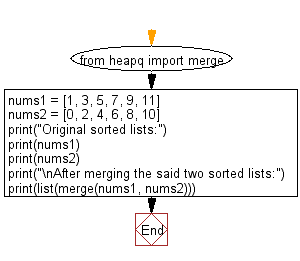﻿ Python heap queue algorithm: Combine two given sorted lists using heapq module - w3resource

# Python: Combine two given sorted lists using heapq module

## Python heap queue algorithm: Exercise-20 with Solution

Write a Python program to combine two sorted lists using the heapq module.

Sample Solution:

Python Code:

``````from heapq import merge
nums1 = [1, 3, 5, 7, 9, 11]
nums2 = [0, 2, 4, 6, 8, 10]
print("Original sorted lists:")
print(nums1)
print(nums2)
print("\nAfter merging the said two sorted lists:")
print(list(merge(nums1, nums2)))
```
```

Sample Output:

```Original sorted lists:
[1, 3, 5, 7, 9, 11]
[0, 2, 4, 6, 8, 10]

After merging the said two sorted lists:
[0, 1, 2, 3, 4, 5, 6, 7, 8, 9, 10, 11]
```

Flowchart:Python Code Editor:

Have another way to solve this solution? Contribute your code (and comments) through Disqus.

What is the difficulty level of this exercise?

Test your Programming skills with w3resource's quiz.

﻿